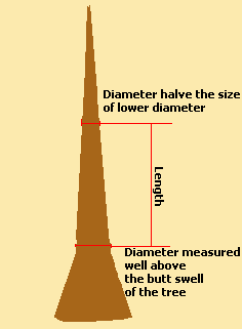Spike's Calculators

# Pressler's Cubic Foot Formula#### A cubic foot formula for estimating tree volume. This formula suits trees whose shape approximates that of a cone or a paraboloid.

The length of the tree is taken from the point where the lower diameter is measured well above the butt swell to a point where the diameter of the log equals one half of the the lower diameter.

Diameter Measured Inside Bark = DIB
Length in Feet ft
DIB Measured Above Butt Swell inch

#### Results:

 Volume in Cubic Feet: ft³